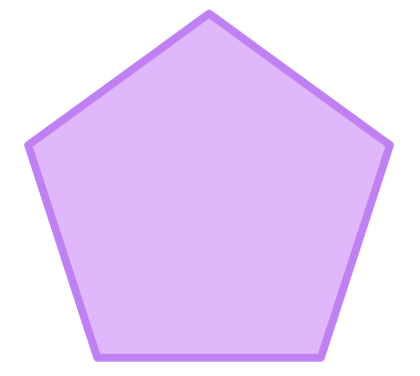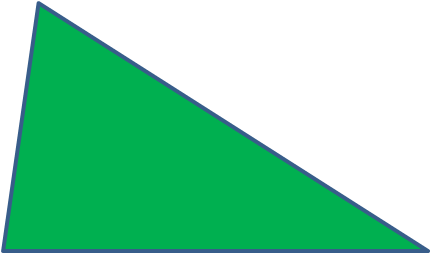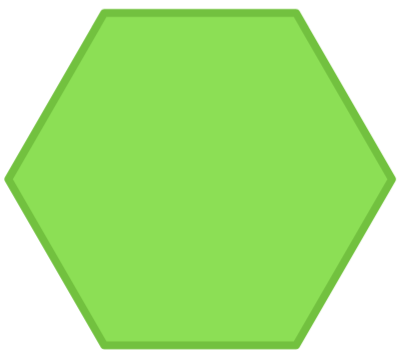# Regular and Irregular Polygons

In this worksheet, students distinguish between regular and irregular polygons based on reasoning about equal sides and angles.Key stage:  KS 2

Curriculum topic:  Maths and Numerical Reasoning

Curriculum subtopic:  2D Shapes: Triangles, Quadrilaterals and Polygons

Difficulty level:### QUESTION 1 of 10

In this worksheet, you must decide whether a polygon is regular or irregular.

The word polygon comes from the Greek language:

polús means many and gõnía means angles.

A polygon is a flat shape with straight sides that are joined to form a closed shape. It will have several angles.

Regular polygons

A regular polygon is one in which all the sides are equal in length and all the interior angles are equal in size.A square has 4 equal sides and 4 equal angles of 90°.A regular pentagon has 5 equal sides and 5 equal angles of 108°.A regular octagon has 8 equal sides and 8 equal angles of 135°.

Irregular polygons

An irregular polygon is one in which either all the sides are not equal in length and/or all the interior angles are not equal in size.This triangle is scalene. It has sides of 3 different lengths and 3 different angles. It is irregular.This pentagon has three 90° angles, but also two angles which are larger. The sides are of differing lengths. It is irregular.

Is the following polygon regular or irregular?An equilateral triangle

regular

irregular

Is the following polygon regular or irregular?An isosceles triangle

regular

irregular

Is the following polygon regular or irregular?A right-angled triangle

regular

irregular

Is the following polygon regular or irregular?regular

irregular

Is the following polygon regular or irregular?regular

irregular

Is the following polygon regular or irregular?A rhombus

regular

irregular

Is the following polygon regular or irregular?A rectangle

regular

irregular

Is the following polygon regular or irregular?regular

irregular

Is the following polygon regular or irregular?A trapezium

regular

irregular

Is the following polygon regular or irregular?A rotated square

regular

irregular

• Question 1

Is the following polygon regular or irregular?An equilateral triangle

regular
EDDIE SAYS
An equilateral triangle has all 3 sides and all 3 angles equal.
• Question 2

Is the following polygon regular or irregular?An isosceles triangle

irregular
EDDIE SAYS
An isosceles triangle only has 2 sides equal and 2 angles equal.
• Question 3

Is the following polygon regular or irregular?A right-angled triangle

irregular
EDDIE SAYS
A right-angled triangle has one angle of 90° and two other smaller angles.
• Question 4

Is the following polygon regular or irregular?regular
EDDIE SAYS
This is a regular hexagon.
• Question 5

Is the following polygon regular or irregular?irregular
EDDIE SAYS
This is an irregular hexagon.
• Question 6

Is the following polygon regular or irregular?A rhombus

irregular
EDDIE SAYS
A rhombus has 4 equal sides, but the angles are not all the same.
• Question 7

Is the following polygon regular or irregular?A rectangle

irregular
EDDIE SAYS
A rectangle has 4 equal angles, but the sides are not all the same.
• Question 8

Is the following polygon regular or irregular?regular
EDDIE SAYS
This is a regular decagon.
• Question 9

Is the following polygon regular or irregular?A trapezium

irregular
EDDIE SAYS
A trapezium does not have 4 equal sides.
• Question 10

Is the following polygon regular or irregular?A rotated square

regular
EDDIE SAYS
A square is regular whether it is rotated or not.
---- OR ----

Sign up for a £1 trial so you can track and measure your child's progress on this activity.

### What is EdPlace?

We're your National Curriculum aligned online education content provider helping each child succeed in English, maths and science from year 1 to GCSE. With an EdPlace account you’ll be able to track and measure progress, helping each child achieve their best. We build confidence and attainment by personalising each child’s learning at a level that suits them.

Get startedStart your £1 trial today.
Subscribe from £10/month.